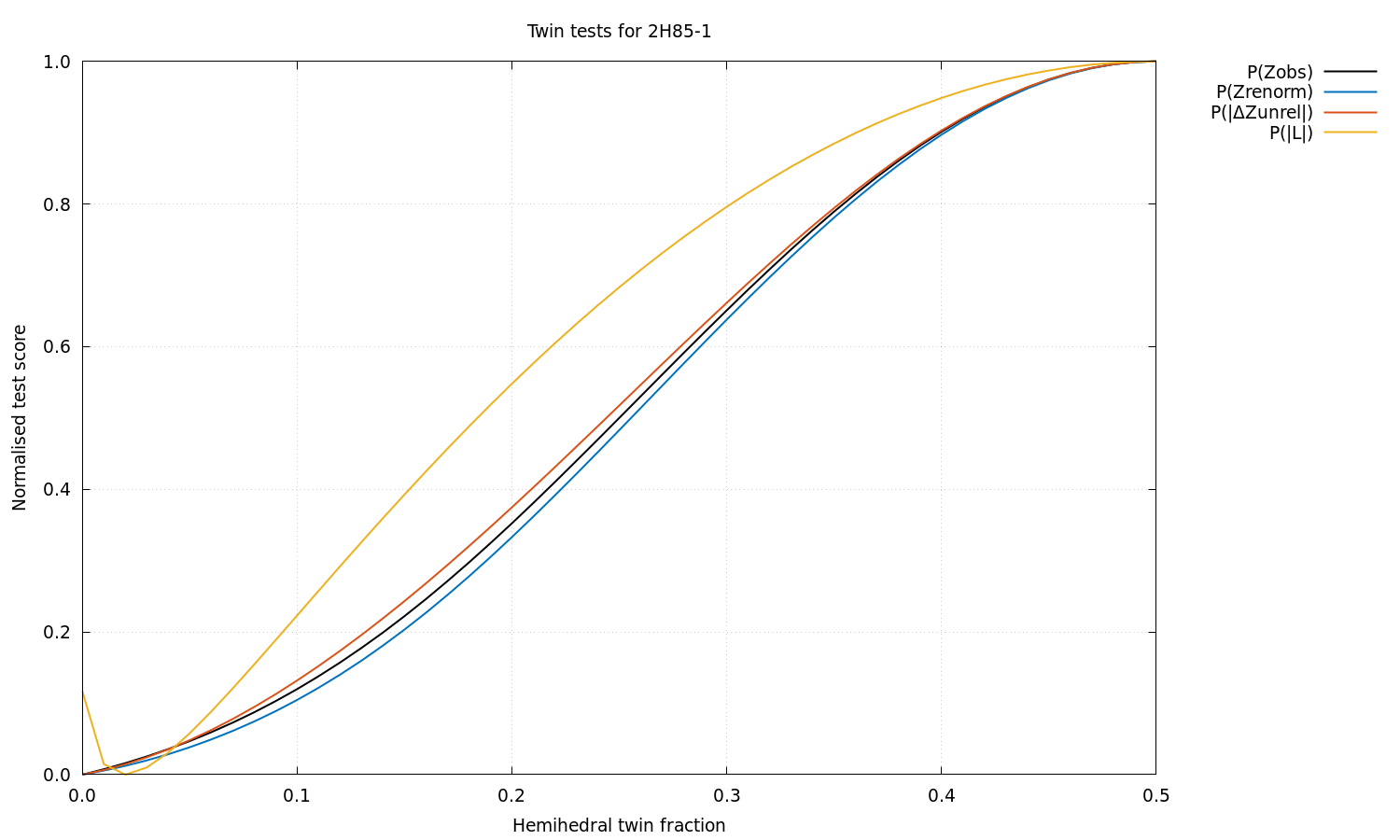### Anisotropy information extracted from the STARANISO log file for 2H85:

```Processing software:          MOSFLM(integration)

Using MTZ column labels:      FP  SIGFP

NOTE: Not doing Bayesian estimation because corrected Is or Fs were input.

Unit cell and space group:      166.570  166.570  166.570  90.00  90.00  90.00   'P 43 3 2'
Nominal diffraction range:       68.002  2.600
Input reflection count:           24881
Unique reflection count:          24880

Diffraction cut-off criterion: Local mean I/sd(I) = 1.20

Diffraction limits & principal axes of ellipsoid fitted to diffraction cut-off surface:

2.574         1.0000   0.0000   0.0000       a*
2.574         0.0000   1.0000   0.0000       b*
2.574         0.0000   0.0000   1.0000       c*

GoF to ellipsoid (d*):  0.0060    Fraction of surface points fitted:  100.0%  (     1619 /     1619)

Number of unobserved reflections inside ellipsoid:        6
Number of observed reflections inside ellipsoid:      24874
Number of observed reflections outside ellipsoid:         0

Lowest cut-off diffraction limit:
2.644 at reflection    0   63    1  in direction   b* + 0.016 c*

Worst diffraction limit after cut-off:
2.643 at reflection    0   63    2  in direction   0.999 b* + 0.032 c*

Best diffraction limit after cut-off:
2.600 at reflection    8   58   26  in direction   0.125 a* + 0.905 b* + 0.406 c*

NOTE that because the cut-off surface is likely to be only very approximately ellipsoidal, in part
due to variations in reflection redundancy arising from the chosen collection strategy, the
directions of the worst and best diffraction limits may not correspond with the reciprocal axes,
even in high-symmetry space groups (the only constraint being that the surface must have point
symmetry at least that of the Laue class).

Fraction of data inside cut-off surface:                          100.0%  (    24874 /    24880)

Fraction of surface truncated by detector edges:                   99.2%  (      709 /      715)

WARNING: Diffraction of the input data has probably been truncated due to an inappropriate
(an)isotropic diffraction cut-off applied in previous processing, or the diffraction pattern may
have extended beyond the edges of the detector.  In the latter case consider the possibilities of
either moving the detector closer or swinging it out, having carefully checked in the former case
that this will not create a risk of spot overlap.

Fraction of total surface above threshold truncated by cusp(s):     0.0%  (        0 /      716)

Eigenvalues & eigenvectors of mean I/sd(I) anisotropy tensor:

4.12         1.0000   0.0000   0.0000       a*
4.12         0.0000   1.0000   0.0000       b*
4.12         0.0000   0.0000   1.0000       c*

Eigenvalues & eigenvectors of weighted CC_1/2 anisotropy tensor:

0.317         1.0000   0.0000   0.0000       a*
0.317         0.0000   1.0000   0.0000       b*
0.317         0.0000   0.0000   1.0000       c*

Eigenvalues & eigenvectors of mean K-L divergence anisotropy tensor:

1.074         1.0000   0.0000   0.0000       a*
1.074         0.0000   1.0000   0.0000       b*
1.074         0.0000   0.0000   1.0000       c*

Eigenvalues & eigenvectors of mean I/E[I] anisotropy tensor:

3.39E-01         1.0000   0.0000   0.0000       a*
3.39E-01         0.0000   1.0000   0.0000       b*
3.39E-01         0.0000   0.0000   1.0000       c*

Ranges of local mean I/sd(I), local weighted CC_1/2, local mean K-L divergence, local mean I/E[I] and D-W factor [= exp(-4 pi^2 s~Us)]:

ISmean  CChalf  KLdive  IEmean  DWfact
0   Grey                     Unobservable*
1   Blue                      Observable*
2   Red|Pink:9    1.20  0.3000   3.244   0.870  0.0658
3   Orange       12.68  0.9737   4.294   1.006  0.1462
4   Yellow       22.33  0.9821   4.789   1.216  0.2827
5   Green        26.65  0.9838   4.927   1.438  0.4763
6   Cyan         29.06  0.9857   5.267   1.598  0.6989
7   Magenta      31.31  0.9879   6.838   1.778  0.8933
8   White        32.54  0.9896   7.692   2.070  0.9947

* Refer to GLOSSARY for explanation of terminology.

The cut-off surface uses a different color scheme:
Unmeasured points are blue (inside the fitted surface) or cyan (outside).
Unobserved points are red (in) or green (out).
Observed points are orange (in) or white (out).
The fitted surface is magenta.

Anisotropic S/N ratio:         0.00    [ = max_h | exp(4 pi^2 s~_h delta(B) s_h) - 1 | <I_h/sd(I_h)> ]

The 'anisotropic S/N ratio', unlike the 'anisotropy ratio' or the 'fractional' anisotropy shown
above, in addition to the anisotropy of the B tensor, takes both the diffraction and the local mean
I/sd(I) into account.

Estimated twin fraction from K-L divergence of observed acentric Z probability (before, after):  0.00  0.00

Estimated twin fraction from K-L divergence of unrelated acentric |delta-Z| probability:         0.00

Padilla & Yeates L test for twinning, acentric moments of |L|:
<|L|> (normal = .500; perfect twin = .375):  0.490
<L^2> (normal = .333; perfect twin = .200):  0.319

Estimated twin fraction from K-L divergence of |L| probability:                                  0.02

```

### Normalised scores from a panoply of hemihedral twinning tests.

For each test, the most likely hemihedral twin fraction based on the data is the one corresponding to the lowest normalised score (i.e. like the R value).  For all the distributional tests (i.e. those of the form P(x)), the raw score before normalisation is the Kullback-Leibler divergence from the theoretical to the observed probability density.  For the non-distributional Britton and Fisher & Sweet tests, it's the count of extra negative intensities obtained by detwinning the data using each possible twin fraction.  Note: click image to enlarge.Reference